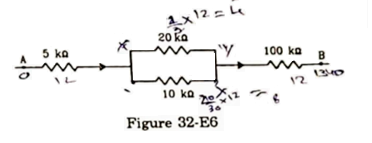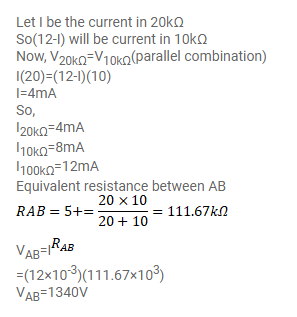# Figure (32-E6) shows a part of a circuit.Question:

Figure (32-E6) shows a part of a circuit. If a current of $12 \mathrm{~mA}$ exists in the $5 \mathrm{k} \Omega$ resistor, find the currents in the other three resistors. What is the potential difference between the points $A$ and $B$ ?Solution: